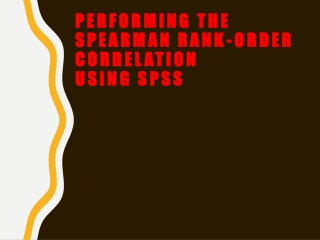DownloadDownload PresentationPerforming the Spearman Rank-Order Correlation Using SPSS

# Performing the Spearman Rank-Order Correlation Using SPSS

Download Presentation## Performing the Spearman Rank-Order Correlation Using SPSS

- - - - - - - - - - - - - - - - - - - - - - - - - - - E N D - - - - - - - - - - - - - - - - - - - - - - - - - - -
##### Presentation Transcript

1. Performing the Spearman Rank-Order CorrelationUsing SPSS

2. OBJECTIVE In this lecture, you will learn the following items: • Performing the Spearman Rank-Order Correlation Using SPSS

3. We will analyze the data from the previous example using SPSS. 1. Define Your Variables First, click the “Variable View” tab at the bottom of your screen. Then, type the names of your variables in the “Name” column. As shown in Figure 1, the first variable is called “Number_of_Visits” and the second variable is called “Mean_Heart_Rate.”

4. 2. Type in Your Values Click the “Data View” tab at the bottom of your screen as shown in Figure 2. Type the values in the respective columns.

5. Analyze Your Data As shown in Figure 3, use the pull-down menus to choose “Analyze,” “Correlate,” and “Bivariate. . . .”

6. Use the arrow button to place both variables with your data values in the box labeled “Variables:” as shown in Figure 4. Then, in the “Correlation Coeficients” box, uncheck “Pearson” and check “Spearman.” Finally, click “OK” to perform the analysis.

7. 4. Interpret the Results from the SPSS Output Window The output table (see SPSS Output 1) provides the Spearman rank-order correlation coefficient (rs = −0.860) labeled Spearman’s rho. It also returns the number of pairs (n = 13) and the two-tailed significance (p approximately 0.000). In this example, the significance is not actually zero. The reported value does not return enough digits to show the significance's actual precision.

8. Based on the results from SPSS, the Spearman rank-order correlation coefficient was significant (rs(11) = −0.860, p < 0.05). Based on these data, we can state that there is a very strong inverse relationship between adult female resting heart rate and the frequency of visits to the gym.

9. Performing the Point-Biserial Correlation Using SPSS We will analyze the data from the previous example using SPSS. 1. Define Your Variables First, click the “Variable View” tab at the bottom of your screen. Then, type the names of your variables in the “Name” column. As shown in Figure 6, the first variable is called “Gender” and the second variable is called “Posttest_Score.”

10. 2. Type in Your Values Click the “Data View” tab at the bottom of your screen as shown in Figure 7. Type in the values in the respective columns. Gender is a discrete dichotomous variable and SPSS needs a code to reference the values. We code male values with 0 and female values with 1. Any two values can be chosen for coding the data.

11. 3. Analyze Your Data As shown in Figure 8, use the pull-down menus to choose “Analyze,” “Correlate,” and “Bivariate. . . .”

12. Use the arrow button near the middle of the window to place both variables with your data values in the box labeled “Variables:” as shown in Figure 9. In the “Correlation Coeficients” box, “Pearson” should remain checked since the Pearson product-moment correlation will perform an approximate point-biserial correlation. Finally, click “OK” to perform the analysis.

13. 4. Interpret the Results from the SPSS Output Window The output table (Output 2) provides the Pearson product-moment correlation coefficient (r = 0.657). This correlation coefficient is approximately equal to the point biserial correlation coefficient. It also returns the number of pairs (n = 17) and the two-tailed significance (p = 0.004).

14. Based on the results from SPSS, the point-biserial correlation coefficient was significant (rpb(15) = 0.657, p < 0.05). Based on these data, we can state that there is a strong relationship between gender and visual detail recognition (as measured by the posttest).

15. SUMMARY The relationship between two variables can be compared with a correlation analysis. If any of the variables are ordinal or dichotomous, a nonparametric correlation is useful. The Spearman rank-order correlation, also called the Spearman’s , is used to compare the relationship involving ordinal, or rank-ordered, variables. The point biserial and biserial correlations are used to compare the relationship between two variables if one of the variables is dichotomous. The parametric equivalent to these correlations is the Pearson product-moment correlation. In this lecture, we explained how to perform the procedures using SPSS.